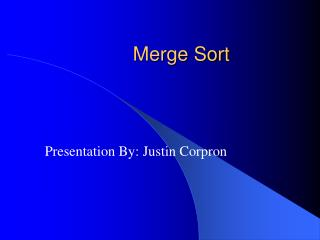# Merge Sort - PowerPoint PPT PresentationDownload PresentationMerge Sort

Merge Sort
Download Presentation## Merge Sort

- - - - - - - - - - - - - - - - - - - - - - - - - - - E N D - - - - - - - - - - - - - - - - - - - - - - - - - - -
##### Presentation Transcript

1. Merge Sort Presentation By: Justin Corpron

2. In the Beginning… John von Neumann (1903-1957) • Stored program • Developed merge sort for EDVAC in 1945

3. Merging • The key to Merge Sort is merging two sorted lists into one, such that if you have two lists X (x1x2…xm) and Y(y1y2…yn) the resulting list is Z(z1z2…zm+n) • Example: L1 = { 3 8 9 } L2 = { 1 5 7 } merge(L1, L2) = { 1 3 5 7 8 9 }

4. Merging (cont.) • The merge Algorithm: From The Art of Computer Programming vol2: Sorting and Searching by Donald E. Knuth (page 160).

5. Merging (cont.) X: Y: Result:

6. Merging (cont.) X: Y: Result:

7. Merging (cont.) X: Y: Result:

8. Merging (cont.) X: Y: Result:

9. Merging (cont.) X: Y: Result:

10. Merging (cont.) X: Y: Result:

11. Merging (cont.) X: Y: Result:

12. Merging (cont.) X: Y: Result:

13. Merging (cont.) X: Y: Result:

14. Divide And Conquer • Merging a two lists of one element each is the same as sorting them. • Merge sort divides up an unsorted list until the above condition is met and then sorts the divided parts back together in pairs. • Specifically this can be done by recursively dividing the unsorted list in half, merge sorting the right side then the left side and then merging the right and left back together.

15. Merge Sort Algorithm Given a list L with a length k: • If k == 1  the list is sorted • Else: • Merge Sort the left side (0 thru k/2) • Merge Sort the right side (k/2+1 thru k) • Merge the right side with the left side

16. Merge Sort Example

17. Merge Sort Example

18. Merge Sort Example

19. Merge Sort Example

20. Merge Sort Example

21. Merge Sort Example Merge

22. Merge Sort Example Merge

23. Merge Sort Example Merge

24. Merge Sort Example Merge

25. Merge Sort Example

26. Implementing Merge Sort • There are two basic ways to implement merge sort: • In Place: Merging is done with only the input array • Pro: Requires only the space needed to hold the array • Con: Takes longer to merge because if the next element is in the right side then all of the elements must be moved down. • Double Storage: Merging is done with a temporary array of the same size as the input array. • Pro: Faster than In Place since the temp array holds the resulting array until both left and right sides are merged into the temp array, then the temp array is appended over the input array. • Con: The memory requirement is doubled. • The merge sort in the text (ch7.6:p238-239) is an example of a double array implementation with Comparable objects.

27. Merge Sort Analysis The Double Memory Merge Sort runs O (N log N) for all cases, because of its Divide and Conquer approach. T(N) = 2T(N/2) + N = O(N logN)

28. Finally… • There are other variants of Merge Sorts including k-way merge sorting, but the common variant is the Double Memory Merge Sort. Though the running time is O(N logN) and runs much faster than insertion sort and bubble sort, merge sort’s large memory demands makes it not very practical for main memory sorting. • Important things to remember for the Midterm: • Best Case, Average Case, and Worst Case = O(N logN) • Storage Requirement: Double that needed to hold the array to be sorted.